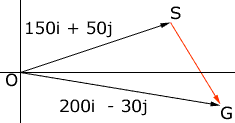Quandaries and Queries Who is asking: Student Level: Secondary Question: In this question, i is a unit vector due east and j is a unit vector due north. An aircraft is flying directly from airport A to airport B, which is 2000 km from A. The velocity in still air of the aircraft is (150i+50j)km/h and the ground speed is (200i-30j)km/h. Calculate (i) the time of flight, to the nearest min (ii) the direction of the wind. Hi, I drew a vector diagram,The vector OS is the velocity in still air and the vector OG is the velocity of the aircraft. The wind must contribute to the velocity being OG so the wind vector must be SG so that OS + SG = OG that is 150i + 50j + ai + bj = 200i - 30 j where SG = ai + bj. Hence ai + bj = (200 - 150)j + (-30 - 50)j Penny Go to Math Central